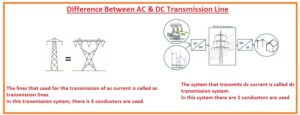Hello, friends, I hope you all are doing great. In today’s tutorial, we will discuss the Difference Between AC & DC Transmission Line.  for ac transmission there is a need of 3 wire that are live wire in some case four wires are used one is neutral and three are phases while dc transmission there is need of 2 conductive wires.

The transmission lines is a system of conductive wires that used to transmit power from generating end to user end.  the transmission lines whose length is eighty kilometers is called short transmission line. The lines that have a length of eight to two hundred kilometers is known as a medium transmission line. While the lines that have a length larger than the one fifty kilometers is called long transmission. The elements of transmission lines are conductors, insulators, clamp, fuses, cross arms, etc. in today’s post, we will have a detailed look at both ac and dc transmission lines with the detailed and find their differences. So let’s gets started with the Difference Between AC & DC Transmission Line.

#### Difference Between AC & DC Transmission Line

AC Transmission Lines

• The lines that used for the transmission of ac current is called ac transmission lines.
• In this transmission system, there is 3 conductors are used.
• This power transmission system consists of inductances and surges.
• The voltage drop during power transmission through this technique is large.
• The uses of breaker are less cost than dc breaker
• If a fault occurs at the powerhouse of ac easy to remove with less cost
• Value of voltage can be changed through the transformer
• In this transmission, there is also skin effect exist.
• This transmission technique required high insulation.
• The dia of conductor used is larger so it is expensive system than dc
• The ac transmission also has interference.
• There is an occurrence of corona loss in this transmission system.
• Reactive power creates different issues in this system
• inductance also make problems in this system
• There is also a dielectric loss that occurs in ac power transmission.DC Transmission

• The system that transmits dc current is called dc transmission system.
• In this system there are 2 conductors are used.
• During this transmission, less voltage loss occurs.
• This system has not surged issues like ac
• it does not has inductance so losses are less
• It does not have signal interference among different communcaiion system
• In this system, there is no existence of skin effect.
• This system required less insulation than the ac system.
• Zero value of dielectric losses
• This system is expensive then dc
• The expense of this system is less than the ac transmission.
• The conductor used in this system has a larger length than the ac transmission.
• If a fault occurs in this system it solution is costly and difficult.
• In this system, there is no use of transformers.
• In this technique, there is the existence of the corona effect.

That is a detailed post about Difference Between AC & DC Transmission Line if you have any queries ask in comments. Thanks for reading. Have a good day.